## A mass of 148 g stretches a spring 13 cm. The mass is set in motion from its equlibrium position with a downward velocity of 10 cm/s and no

Question

A mass of 148 g stretches a spring 13 cm. The mass is set in motion from its equlibrium position with a downward velocity of 10 cm/s and no damping is applied.
(a) Determine the position u of the mass at any time t. Use 9.8 m/s as the acceleration due to gravity. Pay close attention to the units.

in progress 0
2 months 2021-07-31T10:38:16+00:00 1 Answers 2 views 0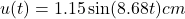0.3619sec

Explanation:

Given that

Mass,m=148 g

Length,L=13 cm

Velocity,u'(0)=10 cm/s

We have to find the position u of the mass at any time t

We know that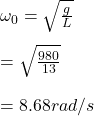Where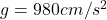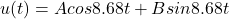u(0)=0

Substitute the value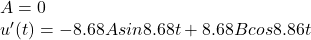Substitute u'(0)=10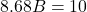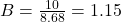Substitute the valuesPeriod =T = 2π/8.68

After half period

π/8.68 it returns to equilibruim

π/8.68 = 0.3619sec